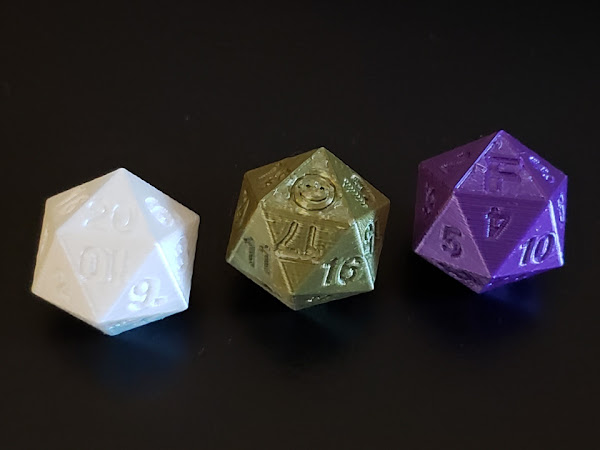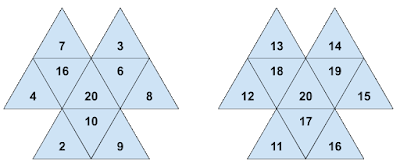### Legitimate uses of loaded dice

This is why, as a game master / dungeon master (DM), I would never allow a player to bring 3D printed dice to a game.

However, I would supply them to players, for certain purposes. In this article I examine the characteristics of "most fair" and "most unfair" designs of d20 dice, which I made in a CAD program and 3D printed for experiments.A fair and balanced d20 (white), a d20 biased to 20 (bronze, with the on the 20 face), and a d20 biased to 1 (purple, with the F for "fail" on the 1 face).

A d20 is a twenty-sided icosahedron with faces numbered from 1 to 20. In the game Dungeons and Dragons, the d20 is ubiquitous. It determines success or failure of an action. It is the first thing rolled any time a player takes an action. The result of the d20 roll, with some modifiers added based on the player's character abilities and skills, determines whether the player's action succeeds or fails, with appropriate consequences.

Before getting into the dice characteristics, I want to describe a simple mechanic that already exists in D&D to simulate a biased d20.

In the fifth edition of the game, there is a mechanism for "advantage" and "disadvantage". In a situation where your character has an advantage or disadvantage, you roll a d20 twice (or roll two of them), and take the higher or lower value, respectively, to determine the outcome of the action.

The probability density function (PDF) of a single fair d20 is flat. Every outcome has equal probability (5%, or 1 in 20). For highest-of-two d20 rolls, the PDF is a uniform sloped line, with a nearly-zero (1 in 400) chance of getting a 1 as the highest of two values (both would have to be 1), and nearly 10% chance (39 in 400) of getting a 20. For lowest-of-two d20 rolls, the probabilities are reversed, but the PDF is still linear between 1 and 20.

Although the game doesn't extend this further, we can. For even greater advantage or disadvantage, a player can roll three d20 dice and pick the highest or lowest value, respectively. The PDF turns out to be a polynomial of order m−1, where m is the number of d20 dice being rolled. When m=1, the polynomial order is 0, which is a constnant, a horizontal line. For two dice (m=2) we get a polynomial of order 1, which is a sloped line. For three dice (m=3) we get a quadratic curve. Rolling three dice, the lowest probability outcome is 1 in 8,000 (1 in 203) and the most probable outcome increases to nearly 15% (1,141 in 8,000).

Where did the 1,141 come from? Well, the probability P(n,m,k) of any particular value k being the maximum value of m rolls of a dn (n-sided die) isn't straightforward or intuitive. The expression is:

$$P(n,m,k) = \frac{1}{n^m} \sum_{i=1}^m (-1)^{i+1}\binom{m}{i} k^{m-i}$$

where the binomial coefficient (I have to write this down because I can never remember it exactly) is:

$$\binom{m}{i} = \frac{m!}{i! (m-i)!}$$

Therefore:

• For two d20 dice, the probability of any value k in the range 1 ≤ k ≤ 20 being the maximum is $$P(20,2,k) = \frac{1}{20^2}(2 k - 1)$$. The chance of rolling a 20 (k=20), then, is $$\frac{39}{400}$$.
• For three d20 dice, the probability of any value k in the range 1 ≤ k ≤ 20 being the maximum is $$P(20,3,k) = \frac{1}{20^3}(3 k^2 - 3 k + 1)$$. In this case, the probability for k=20 is $$\frac{1,141}{8,000}$$.

Simulating 10,000 trials, the observed outcomes match the polynomial curves quite well:

## Fair vs unfair d20

So, what is a "fair" d20?

Physical characteristics play an important role, of course: a fair die is a balanced die, with good symmetry, constant density, uniform thickness between opposing faces, corners and edges uniformly sharp or rounded.

It is also possible for a d20 to be balanced numerically, not just by making sure opposite faces all add up to 21, but also by balancing the distribution of outcomes around each face and vertex.

A company called MathArtFun makes a large variety of dice with any number of faces ranging from 3 to 120. They spent some effort making their d20 numerically balanced, to ensure a uniform distribtion of outcomes.

A numerically-balanced d20 has these characteristics:

• Each pair of opposite faces adds up to 21 (as you would expect for any d20).
• The five faces touching each vertex add up to 52 or 53 (average 52.5).
• The 3 faces adjacent to any face add up to 31 or 32 (avg 31.5).

These conditions result in a d20 that provides a fair distribution of outcomes even when there are imperfections in shape and balance.

How about a unbalanced d20 that takes advantage of physical imbalance as well as numerical imbalance? Well, obviously:

• The d20 appears symmmetrical and perfectly shaped, but internally it is heavier on one side.
• All the high numbers are on the lighter side if the d20 is biased high, or on the heavier side if the d20 is biased low.
• The "20" face corresponds to the lightest or heaviest face, depending on the bias.
• The 20 is surrounded by the next highest numbers, 17, 18, and 19.
• The remaining numbers 11, 12, 13, 14, 15, and 16 surround the four highest numbers. One can attempt to distribute them in a balanced way, but there's no point, really.
• Each pair of opposite faces adds up to 21, just like the numerically balanced d20.
• And why not? If the large numbers are on one half of the icosahedron, and the small numbers are on the other half, they may as well have this opposing balance.
• It gives an illusion of fairness, too.
• This also simplifies mapping the faces in software because you need only to define the locations of half of them, and the other half are automatically determined.

Here is how the face maps look for a balanced and unbalanced d20. Half of the icosahedron is shown unwrapped and flattened out, with the 20 on the center face.Face map of a numerically balanced d20 (left) and a deliberately unbalanced d20 (right). Only one half of the d20 icosahedron is shown, unpeeled and flattened. The opposite face values are such that each pair of opposite faces adds up to 21.

## Making the dice

I used OpenSCAD to design three d20s for 3D printing. These are pictured at the top of this article: fair-and-balanced, biased to high scores, and biased to low scores. I made the OpenSCAD model as well as STL files (available on Printables or Thingiverse_.

The fair d20 isn't completely solid; for faster printing I used a gyroid infill at 30%. A gyroid is a 3D surface that provides structural integrity in all three dimensions, not just one, so it's reasonably balanced. Because it isn't solid, it's about 5 grams in weight, just enough heft for throwing.

The unfair dice are designed with a half-icosahedron hollowed out from the inside. The high-biased d20 has the high numbers engraved around the hollow half, and the low-biased d20 has the low numbers engraved around the hollow half. The infill of the non-hollow side is also gyroid, but at a 60% density to have a total mass comparable to the fair d20.

## Testing

I actually started writing this article seven months ago, but couldn't publish it because I wanted to test my dice. Well, that is, I wanted them to be tested, but there was nobody to do it but me. And rolling dice hundreds of times is an exercise in tedium. It actually took me seven months to get it done, testing occasionally when I felt like it.

This was my procedure:

1. Find a billard table. It has a nice, uniform, level surface, not too hard, not too soft.

2. Roll the three d20s and record the results in a spreadsheet.
• Try to roll consistently, letting the dice roll out of my hand from about 12 inches above the table.
• Re-roll a d20 before recording the result if anything interferes with it (hitting the edge of the table, another d20, or my laptop on the table)
3. Repeat from step 2 until collecting at least six occurrences of every outcome from 1 to 20 on all three dice.

I never realized how long it would take to complete that last requirement. Getting more than six occurrences of every value took about 470 trials, so I rounded it up to 500. That's far fewer than the 10,000 simulated trials used to plot the graph above. Only 500 trials isn't enough to smooth out the data, but even with the large variations in outcomes for each d20, one can definitely see trends:

• The fair d20 outcomes are reasonably flat with an average roll of 10.2, near the expectation of 10.5 (arithmetic average of the integers 1 though 20).
• The unfair high-bias d20 is definitely biased high, with an average roll of 13.9.
• The unfair low-bias d20 is definitely biased low, with an average roll of 7.7.

My unfair dice don't appear to have a linear distribution of outcomes, however, unlike the "advantage" or "disadvantage" mechanic described above when rolling two dice. There isn't really enough data to tell, but it does look like they're nonlinear, possibly a quadratic or cubic shape, closer to what one gets when one rolls three times and picks the higest or lowest. The graph above shows a least-squares-fit quadratic function overlaid on the data points for the unfair d20s.

Because it's so easy to 3D-print an unfair d20, no self-respecting game master should allow a player to bring one into the game. They could be offered to players, though.

You can download STL models for these three d20s from Printables or Thingiverse.

1.cool man, you did so much work for this lol. This is honestly really cool

2.Upmost respect to you for making probabilities actually interesting.

3.This was really a fun read. I was intrigued enough to look at my D20s & find that none of them are numerically balanced. (this won't stop me from using them though!)

4.A little mathematical hocus-pocus: a loaded roll dN can be added to the result of other rolls dN, and the result modulo N will be at least as fair as the fairest dN in the bunch. The full proof is a task for a better mathematician than I, but the seed of proof is intuitive (given one knows modular arithmetic): the probability distribution of a fair roll plus some arbitrary number just has all the labels switched; it hasn't actually changed the distribution.

This allows you as a referee to offer a Faustian bargain to the theoretical rapscallion who somehow wishes to supply a d20 of dubious bias while also understanding and consenting to the above procedure: perhaps you will add the result of a fair d20 and the result will be fair no matter what... or perhaps you will add any number of your choosing or any roll of your choosing in assuming the d20 in question is fair... (blackjack!).

1.I can't say I agree without seeing the proof, because intuitively, in an extreme case where a loaded d20 rolls 20 almost always, adding it to any other roll of the same d20 modulo 20 doesn't really change the biased outcome of the loaded d20. Same if the d20 always rolls 1; adding 1 to it modulo 20 results in 2, still a biased low roll.